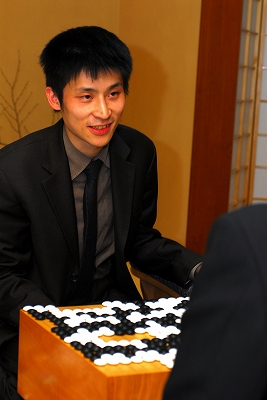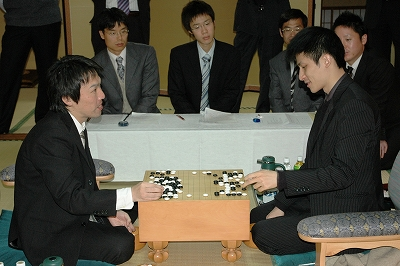Posts Tagged ‘yamada kimio’

Cho U Keeps Oza Title

Thursday, November 26th, 2009

Cho U defeated challenger Yamada Kimio with 3 straight wins and kept his Oza title for another year.The 3rd game record is here. Cho U (white) won by 3.5 points.

(; KM[6.5] EV[57th Oza title match 3] FF SZ GM PW[Cho U] WR[Oza] DT[2009-11-26] BR[9d] RE[W+3.5] PB[Yamada Kimio] ;B[pd];W[dd];B[pq];W[dq];B[do];W[cm];B[cq];W[gq];B[dp];W[dr];B[bo] ;W[cj];B[ip];W[cr];B[gp];W[fp];B[fo];W[ep];B[eo];W[hp];B[go];W[hq] ;B[ho];W[iq];B[jp];W[jq];B[kp];W[lq];B[ch];W[ej];B[eh];W[gc];B[gi] ;W[cf];B[ic];W[gk];B[ie];W[qf];B[qh];W[kc];B[ge];W[nc];B[pc];W[ph] ;B[pi];W[pg];B[qj];W[ne];B[re];W[qe];B[rd];W[nh];B[dl];W[el];B[bd] ;W[cc];B[ce];W[de];B[bf];W[dm];B[po];W[qm];B[qo];W[ri];B[qi];W[nj] ;B[pl];W[nl];B[pm];W[fh];B[fi];W[ei];B[eg];W[ff];B[fg];W[gf];B[ke] ;W[cd];B[be];W[ef];B[bi];W[ig];B[md];W[nd];B[lb];W[ob];B[rf];W[jb] ;B[lc];W[ib];B[kd];W[lf];B[jg];W[le];B[ld];W[jf];B[hg];W[jh];B[hf] ;W[gg];B[kg];W[if];B[hh];W[kf];B[gh];W[hc];B[id];W[bq];B[hl];W[gl] ;B[hm];W[jj];B[mr];W[lr];B[kl];W[cg];B[bg];W[bj];B[bc];W[bb];B[ab] ;W[cb];B[fd];W[fc];B[lk];W[hk];B[ki];W[ji];B[ai];W[il];B[im];W[jl] ;B[jm];W[or];B[mi];W[ni];B[mq];W[lp];B[np];W[ko];B[ln];W[lo];B[mn] ;W[no];B[mo];W[mp];B[nq];W[kn];B[km];W[nn];B[ml];W[ol];B[kh];W[pk] ;B[qk];W[on];B[mm];W[pn];B[ok];W[nk];B[qn];W[jn];B[mg];W[mh];B[lg] ;W[ng];B[mf];W[me];B[in];W[oj];B[ql];W[ms];B[ns];W[ls];B[ih];W[pb] ;B[qb];W[rb];B[qc];W[qa];B[rc];W[qg];B[rg];W[kj];B[lj];W[hj];B[nr] ;W[op];B[oq];W[oo];B[pp];W[cp];B[co];W[fm];B[gm];W[fn];B[bm];W[bl] ;B[am];W[bp];B[ao];W[pe];B[ed];W[ec];B[kq];W[kr];B[io];W[kb];B[ka] ;W[je];B[jd];W[ac];B[ad];W[ci];B[dh];W[df];B[na];W[nb];B[bh];W[kk] ;B[oi];W[nf];B[li];W[pj];B[di];W[dj];B[fj];W[fk];B[al];W[ak];B[aj] ;W[bk];B[cn];W[ja];B[la];W[ra];B[sb];W[oh];B[en];W[em];B[ap];W[aq] ;B[od];W[oe];B[mk];W[ba];B[gn])

Complete tournament table is here.

Cho U – Yamada Kimio 2:0 in Oza Title

Wednesday, November 25th, 2009

Cho U, Oza title holder, won the second game as well against challenger Yamada Kimio. Cho U is just one win away from keeping this title for another year.The game record is here. Cho U (black) won by resignation.

(; DT[2009-11-24] PB[Cho U] RE[B+R] PW[Yamada Kimio] FF SZ GM BR[Oza] KM[6.5] EV[57th Oza title match 2] WR[9d] ;B[pd];W[dd];B[qp];W[dp];B[fq];W[hq];B[nq];W[qj];B[cn];W[fp];B[cq] ;W[dq];B[cp];W[do];B[cr];W[dr];B[fc];W[cf];B[db];W[qf];B[nd];W[ic] ;B[cc];W[lc];B[ld];W[md];B[me];W[mc];B[ne];W[kd];B[qe];W[qm];B[nc] ;W[rf];B[le];W[cl];B[dn];W[ci];B[jd];W[jc];B[ke];W[id];B[kc];W[kb] ;B[pm];W[ql];B[iq];W[ip];B[jp];W[hp];B[jr];W[jo];B[hr];W[kp];B[jq] ;W[mq];B[ko];W[np];B[oq];W[lp];B[gq];W[gp];B[eo];W[ep];B[jn];W[io] ;B[kn];W[lr];B[fs];W[cs];B[mo];W[mp];B[on];W[co];B[nr];W[po];B[oo] ;W[bo];B[qn];W[rn];B[qo];W[el];B[hm];W[rd];B[fm];W[em];B[be];W[ce] ;B[di];W[dh];B[ei];W[cj];B[oj];W[ph];B[pk];W[qk];B[pg];W[gk];B[ik] ;W[qh];B[bf];W[bg];B[oh];W[ii];B[hj];W[hh];B[cd];W[jj];B[jk];W[lj] ;B[kk];W[pl];B[ol];W[nk];B[ok];W[ni];B[oi];W[af];B[ie];W[gd];B[de] ;W[df];B[ee];W[gc];B[ef];W[eh];B[fh];W[gg];B[eg];W[ch];B[hi];W[ih] ;B[kj];W[ki];B[ge];W[fe];B[ff];W[he];B[fd];W[hf];B[rm];W[ro];B[rp] ;W[rl];B[qc];W[lh];B[dg];W[cg];B[lk];W[mk];B[mj];W[nj];B[li];W[mi] ;B[lj];W[mg];B[ml];W[rc];B[qb];W[nb];B[re];W[se];B[ob];W[fb];B[so] ;W[sm];B[bd];W[fg];B[fj];W[gf];B[dc];W[fe];B[ed];W[eb];B[ec];W[fn] ;B[dk];W[dj];B[ek])

The next game will be played on November 26th. Complete tournament table is here.

Cho U Wins First Game in Oza Title Match

Friday, October 30th, 2009

Cho U, title holder of the Oza title, defeated challenger Yamada Kimio in the first game of this best-of-five title match.

This title must be quite important for Cho U: while he is still dominating the Japanese Go by the number of title he is holding, his very recent loss of Meijin title to Iyama Yuta is a sign that Cho U may not be in top shape anymore.The game record is here.

(; EV[57th Oza title match 1] KM[6.5] FF SZ GM PW[Cho U] WR[Oza] DT[2009-10-23] BR[9d] RE[W+R] PB[Yamada Kimio] ;B[qd];W[dc];B[dp];W[pp];B[ce];W[ed];B[ci];W[oc];B[kd];W[pe];B[qe] ;W[pf];B[qg];W[qc];B[pg];W[pd];B[ng];W[ic];B[rc];W[qb];B[qn];W[nq] ;B[rp];W[kq];B[qq];W[pm];B[pn];W[om];B[qm];W[on];B[fq];W[bp];B[cp] ;W[bo];B[bq];W[br];B[cq];W[cl];B[bm];W[cm];B[bn];W[co];B[cn];W[do] ;B[dn];W[eo];B[gp];W[go];B[ho];W[em];B[dm];W[dl];B[bl];W[fp];B[hq] ;W[aq];B[cr];W[hn];B[io];W[ej];B[en];W[eq];B[ep];W[fo];B[er];W[fm] ;B[el];W[fl];B[ek];W[dj];B[fk];W[gk];B[fj];W[cj];B[bj];W[bi];B[ck] ;W[dk];B[bk];W[ch];B[fn];W[gn];B[gm];W[gl];B[hm];W[il];B[im];W[in] ;B[jm];W[jn];B[di];W[ei];B[dh];W[dg];B[eh];W[fi];B[gj];W[fh];B[ik] ;W[hj];B[gi];W[jl];B[km];W[gh];B[hi];W[ij])

The next game will be played on November 24th. Complete tournament table is here.